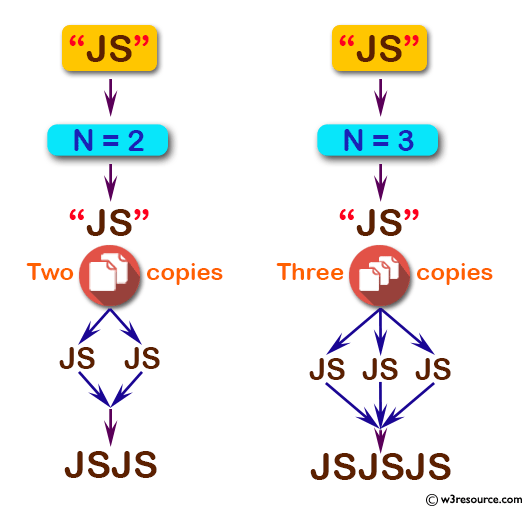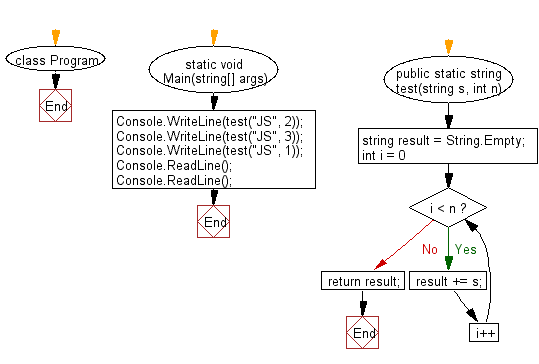﻿ C# - Create a new string which is n copies of a given string# C# Sharp Basic Algorithm Exercises: Create a new string which is n copies of a given string

## C# Sharp Basic Algorithm: Exercise-25 with Solution

Write a C# Sharp program to create a new string which is n (non-negative integer ) copies of a given string.

Pictorial Presentation:Sample Solution:-

C# Sharp Code:

``````using System;
namespace exercises
{
class Program
{
static void Main(string[] args)
{
Console.WriteLine(test("JS", 2));
Console.WriteLine(test("JS", 3));
Console.WriteLine(test("JS", 1));

}
public static string test(string s, int n)
{
string result = String.Empty;
for (int i = 0; i < n; i++)
{
result += s;
}
return result;
}
}
}
```
```

Sample Output:

```JSJS
JSJSJS
JS```

Flowchart:C# Sharp Code Editor:

Improve this sample solution and post your code through Disqus

What is the difficulty level of this exercise?

Test your Programming skills with w3resource's quiz.

﻿